# Orbital Quantities of Satellitesa program for the Sharp EL-9600, EL-9650and EL-9900 graphing calculators

### Earth Centred Circular Motion of Artificial Satellites

• What is the orbital radius of a GPS-satellite which makes one revolution within 11.967 h?
• A geostationary satellite rotates once in 23 h and 56 min (23.93447 h), just like the earth itself. What is the altitude of the satellite above the equatorial surface? What is the orbital velocity of a geostationary satellite?
• During an interval of several months the mean orbital altitude of the International Space Station (ISS) varied between 330 km and 410 km. What are the corresponding periods?
• Within a few years the Galileo system, Europe's satellite positioning system, will be fully operational with 30 satellites positioned in three circular orbits at altitudes of 23616 km above the earth's surface. What is the expected period? What the angular velocity?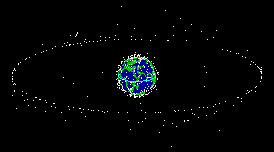Artificial satellites in orbit (27-11-2004)
(source: science.nasa.gov/Realtime/..)

Find answers to questions like those above. Start up the program, select one of the following six orbital quantities and put in a value.
T, the period of the orbiting satellite (h, hour)
h, the altitude (height) of the satellite above the equatorial surface of the earth (km)
v, the orbital velocity (m/s)
a, the centripetal acceleration (m/s²)
ω, the angular velocity (°/min, degree/minute)

From the given value your Sharp-calculator computes the remaining five quantities.
In addition some other quantities are displayed:
ve, the escape velocity (m/s);
Ekin, the kinetic energy (MJ/kg);
Epot, the potential energy with reference to the infinite (MJ/kg);
ΔEeq, the difference in total mechanical energy per kilogram between the orbiting mass and a mass fixed at the equatorial surface (MJ/kg).

### An Illustrated Example

To take photographs of the earth's surface, a polar satellite has been put into orbit at an altitude of 800 km above the equatorial surface (thus having an orbital radius of 6378.137 km + 800 km). What is the period, what the velocity, the acceleration, etc?
Select "2:Height h (km)" in the displayed menu (see the first picture below). Then the altitude is required (picture not shown). Key in 800 and press Enter.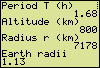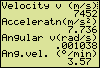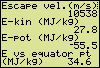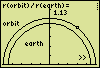(TI images. The screen examples
differ slightly from Sharp pictures)

The next screen shows a period of 1.68 h, so one revolution lasts about 101 min. The orbital radius is displayed twice, once in km and once it is expressed as a number of earth radii. Likewise, the angular velocity is shown in the units rad/s and °/min.

### Special Input

Feel free to put in an expression. For instance, if in an article on the International Space Station a period of 91.97 min is reported, the expression 91.97/60 is the most plausible input (in the unit hour). Similarly, convert an angular velocity of 0.00117 radian/second into °/min by multiplying it by the conversion factor 60*180/π. The factor is stored in memory X, so feed in either .00117*60*180/π or .00117X.

### Value of 'Big G'

Nowadays Newton's gravitational constant G is a near four significant figures accurate quantity: 6.67428 (67) × 10–11 N m2 kg–2. The more accurate value of G·Mearth is adopted in the program "SATELLIT".
G = (6.67428 ± 0.00067)·10–11 N m2 kg–2;
μ = GMearth = (3.986004418 ± 0.000000008)·1014  N m2 kg–1
• Earth mass = Mearth = 5.972·1024 kg
Sources:
http://physics.nist.gov/cuu/;
http://en.wikipedia.org/wiki/Gravitational_constant;
http://www.nineplanets.org (*).

After running the program, the value of GM is found in memory G.

(*) According to a new definition (International Astronomical Union, Prague, 24-08-2006) the Solar System consists of only eight planets, that is without Pluto. A planet is defined as a celestial body that (a) is in orbit around the Sun, (b) has sufficient mass for its self-gravity to overcome rigid body forces so that it assumes a hydrostatic equilibrium (nearly round) shape, and (c) has cleared the neighbourhood around its orbit. From now on Pluto is a member of a new class of objects called "dwarf planets".

### Influence of Sun and Moon

Gravitational forces in consequence of the presence of the sun and the moon are not taken into account. In lower orbits their influence is negligible. The resulting force of both sun and moon is maximal when the celestial bodies are in conjunction and at the same time their distances from earth are minimal. With an orbital radius less than 25 Mm the maximal resulting force will not exceed 1 % of the gravitational force of the earth (and will not exceed 5 % with radii less than 56 Mm). Fluctuations in the total force exerted on an orbiting artificial satellite remain below 1 % up to an orbital radius of 93 Mm (and below 5 % with a radius below 187 Mm).

### Program Listing

[ Remarks ]
SATELLIT

Rem MEM AEGHNRTVWXZ
Rem 2000 VER2009
[ Memories, program version ]
[ > 2ndF,PRGM > PRGM > Rem ]
ClrT
[ > 2ndF,PRGM > SCRN > ClrT ]
Print "** SATELLITE **"
Print "
Print "SETTINGS REMINDER"
Print "
Print "FLOATPT (SET UP, FSE)"
Print "PLOTOFF (STAT PLOT)"
Print ">>"
[ > MATH > INEQ > ">" ]
Wait
ClrT
Print "** SATELLITE **"
Print "
Print "EARTH CENTRED"
Print "CIRCULAR MOTION"
Print "INFO:"
Print "WWW.TENHORN.COM"
Print ">>"
Wait :ClrT
Print "** SATELLITE **"
Print "
Print "SELECT A KNOWN"
Print "ORBITAL QUANTITY."
Print "ALL OTHERS WILL BE"
Print "CALCULATED."
Print ">>"
3.986004418*10^14→G
[ ^ : ab key ]
[ *10^14 or E14 ]
[ → : STO key ]
6378137→E
60*180/π→X
Label IN
Wait :ClrT
Print "1 PERIOD T (H)"
Print "2 HEIGHT H (KM)"
Print "4 VELOCITY V (M/S)"
Print "5 ACCELERATION (M/S2)"
Print "6 ANGULAR VEL. (°/MIN)"
Print "ENTER NUMBER (0:STOP)"
Input N
ClrT
Print "ENTER VALUE OF:"
Print "
If N=1 Goto IT
If N=2 Goto IH
If N=3 Goto IR
If N=4 Goto IV
If N=5 Goto IA
If N=6 Goto IW
Print "NONE. PRESS CL."
End
Label IT
Print "PERIOD T (HOUR)"
Input T:3600T→T
(G/4/π^2*T^2)^(1/3)→R
Goto R
Label IH
Print "ALTITUDE H (KM)"
Input H:1000H→H:H+E→R
Goto R
Label IR
Print "(KM)"
Input R:1000R→R
Goto R
Label IV
Print "ORBITAL VELOCITY V"
Print "(M/S)"
Input V
max(abs(V),1)→V
G/V^2→R
Goto R
Label IA
Print "CENTRIPETAL"
Print "ACCELERATION A"
Print "(M/S2)"
Input A
max(abs(A),10^-15)→A
(G/A)^.5→R
Goto R
Label IW
Print "ANGULAR VELOCITY W"
Print "(°/MIN)"
Input W:W/X→W
(G/W^2)^(1/3)→R
Label R
If R≥E Goto R2
ClrT
Print "AN ORBIT BELOW THE"
Print "EQUATORIAL SURFACE."
Print "INTERVENTION:"
Print "ALTITUDE = 0 KM"
Print E/1000:Print ">>"
Wait
Label R2
max(R,E)→R
(G/R^3)^.5→W:2π/W→T
R–E→H:(G/R)^.5→V
G/R^2→A
ClrT
Print "PERIOD (H)"
Print round(T/3600,2)
Print "ALTITUDE (KM)"
Print round(H/1000,0)
Print round(R/1000,0)
Print round(R/E,2)
Wait :ClrT
Print "VELOCITY (M/S)"
Print round(V,0)
Print "ACCELERATION (M/S2)"
Print round(A,3)
Print round(W,6)
Print "ANGULAR VELOC. (°/MIN)"
Print round(WX,2)
Wait :ClrT
Print "ESCAPE VELOCITY (M/S)"
Print round(2^.5*V,0)
Print "KINET. ENERGY (MJ/KG)"
Print round(G/R/2/10^6,1)
Print "POT. ENERGY (MJ/KG)"
Print round(-G/R/10^6,1)
Print "E VS EQUATORPT (MJ/KG)"
Print round((-G/R/2–E^2*2.658747/10^9+G/E)/10^6,1)
Wait
DrawOFF :Rect
1.02→Z:-Z→Xmin
Z→Xmax:.2→Xscl
0→Ymin:.2→Yscl
Z→Ymax
ClrDraw
Circle(0,0,E/R)
Circle(0,0,1)
Circle(.6,.8,.024)
Text(0,2,"ORBIT")
Text(8,max(5,ipart ((1E/R)*9.7)),"EARTH")
Text(25,9,">>")
Goto IN

Before starting the program, possible active statistical plots have to be disabled with the "PlotOFF" command.
PlotOFF is not programmable. At the normal screen press:
> 2ndF, STAT PLOT > ON/OFF > PlotOFF.

If you need help with programming your calculator, this exercise will put you on the way: Creating a Program for the Sharp EL calculator

Hein ten Horn
Top | Programs Sharp EL | Home